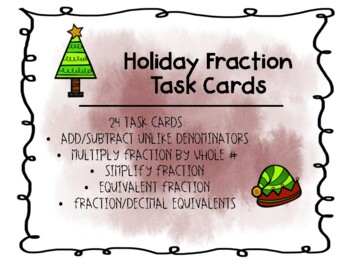# Holiday Fraction Task Cards - VA Math SOL 5.2Subject
Resource Type
File Type

PDF

Product Rating
Standards
Also included in:
1. These resources are made under VA 5th Grade SOLs and have a holiday theme! Resources Included - - Holiday Cookies Dichotomous Key- Holiday Cupcakes Dichotomous Key - Create Your Own Holiday Elf Dichotomous Key- Compare/Order Fractions, Decimals, & Mixed Numbers Holiday Task Cards- Holiday Fracti
\$6.50
\$4.50
Save \$2.00
2. This bundle includes activities and skills dealing with fractions. Skills included -- Add/Subtract Mixed Numbers Exit Tickets- Fraction & Decimal Equivalent Go Fish - Fraction Computation Word Problems - Holiday Fraction Task Cards- Ordering Fractions Exit Ticket If you prefer, you can purchase
\$5.75
\$4.00
Save \$1.75
• Product Description
• StandardsNEW

This set of 24 task cards practices the following VA 5th Grade SOLs (5.2b)

- Multiplying Fractions by Whole Numbers

- Simplify Fractions

- Equivalent Fractions

- Fraction/Decimal Equivalents

Solve real world problems involving multiplication of fractions and mixed numbers, e.g., by using visual fraction models or equations to represent the problem.
Apply and extend previous understandings of multiplication to multiply a fraction or whole number by a fraction.
Solve word problems involving addition and subtraction of fractions referring to the same whole, including cases of unlike denominators, e.g., by using visual fraction models or equations to represent the problem. Use benchmark fractions and number sense of fractions to estimate mentally and assess the reasonableness of answers. For example, recognize an incorrect result 2/5 + 1/2 = 3/7, by observing that 3/7 < 1/2.
Add and subtract fractions with unlike denominators (including mixed numbers) by replacing given fractions with equivalent fractions in such a way as to produce an equivalent sum or difference of fractions with like denominators. For example, 2/3 + 5/4 = 8/12 + 15/12 = 23/12. (In general, 𝘢/𝘣 + 𝘤/𝘥 = (𝘢𝘥 + 𝘣𝘤)/𝘣𝘥.)
Solve word problems involving multiplication of a fraction by a whole number, e.g., by using visual fraction models and equations to represent the problem. For example, if each person at a party will eat 3/8 of a pound of roast beef, and there will be 5 people at the party, how many pounds of roast beef will be needed? Between what two whole numbers does your answer lie?
Total Pages
Included
Teaching Duration
N/A
Report this Resource to TpT
Reported resources will be reviewed by our team. Report this resource to let us know if this resource violates TpT’s content guidelines.